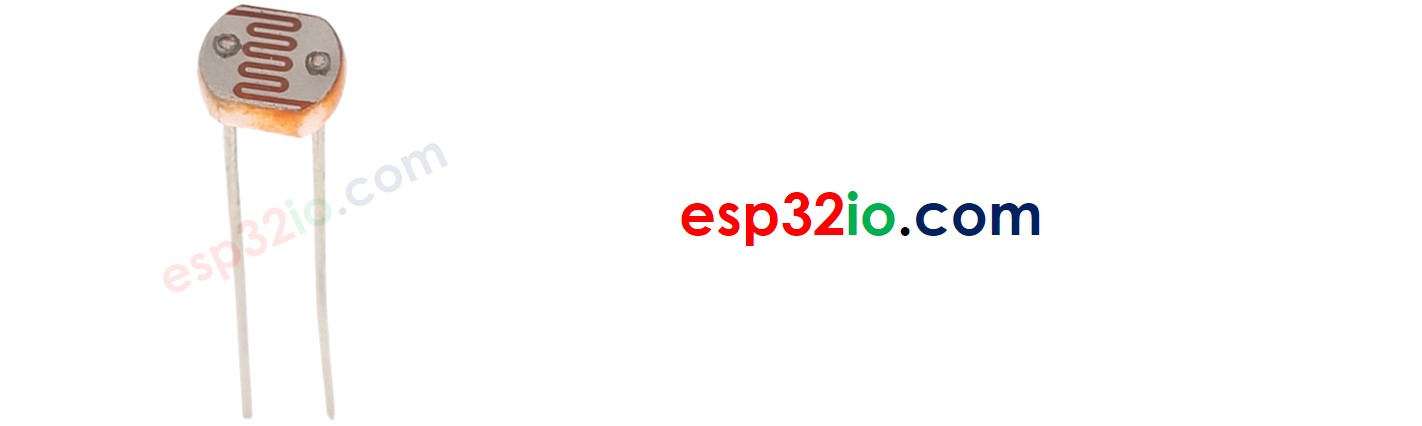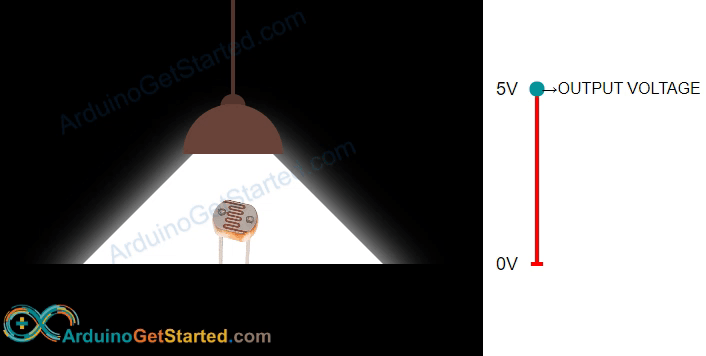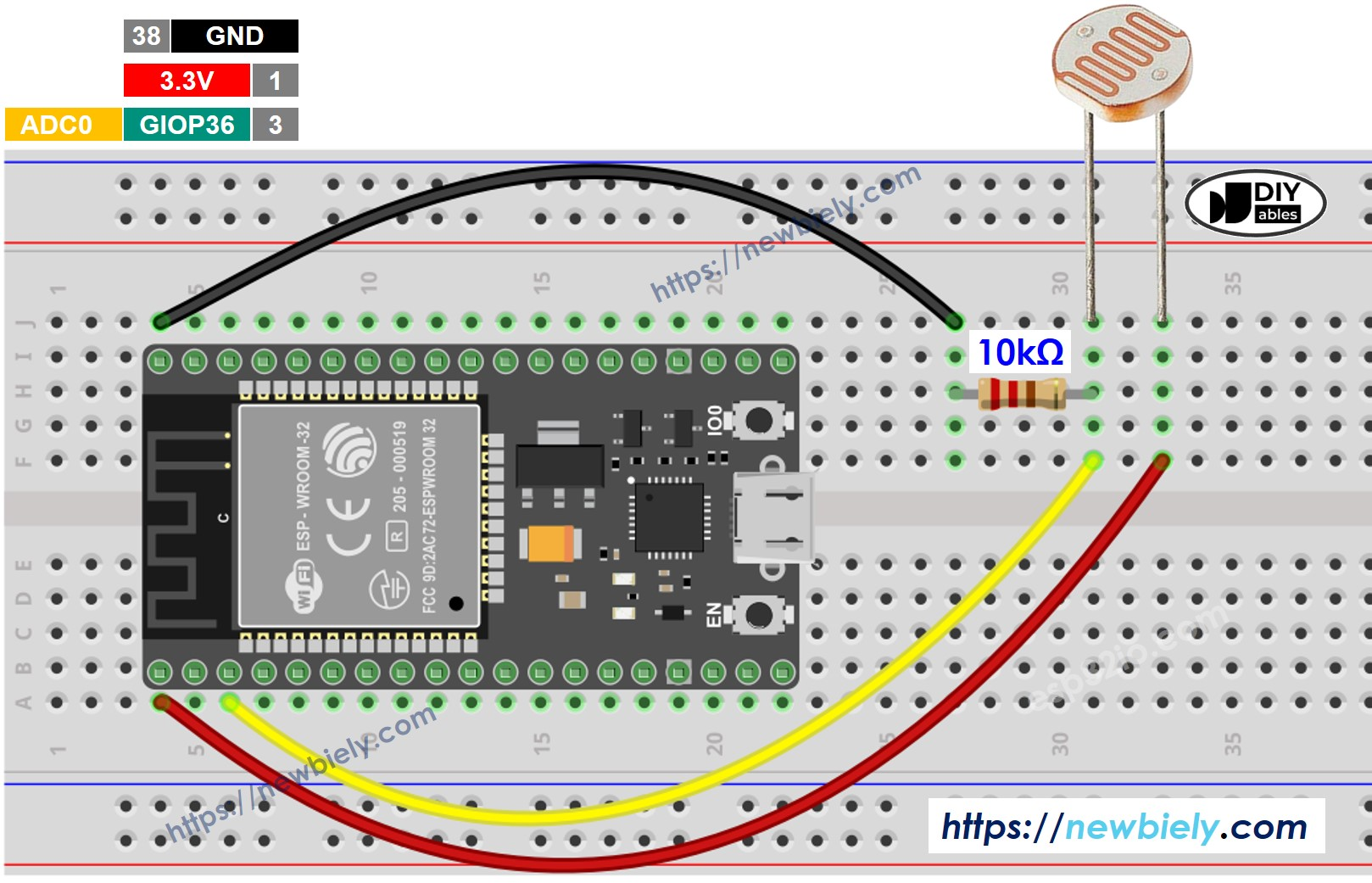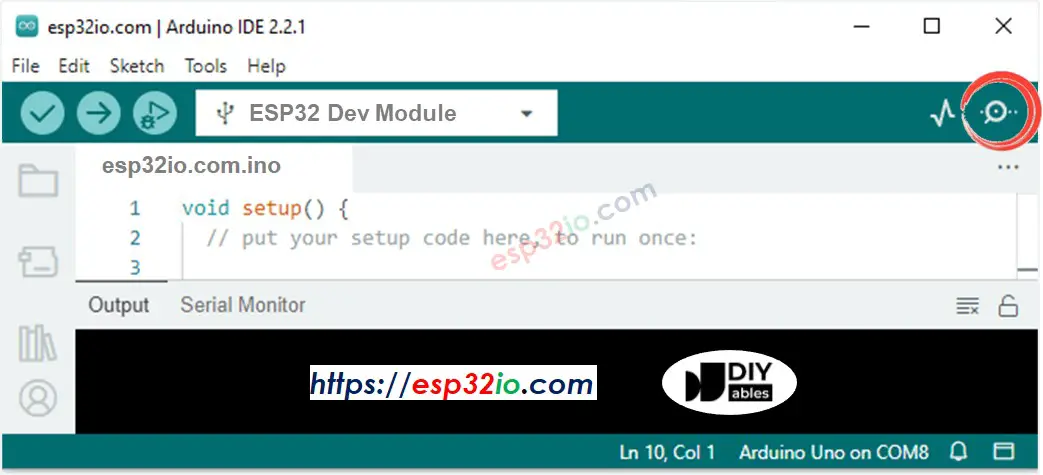# ESP32 - Light Sensor

## Hardware Required

 1 × ESP-WROOM-32 Dev Module 1 × Micro USB Cable 1 × Light Sensor 1 × 10 kΩ resistor 1 × Breadboard n × Jumper Wires
Please note: These are affiliate links. If you buy the components through these links, We may get a commission at no extra cost to you. We appreciate it.

The light sensor used in this tutorial is a photoresistor, which is also called light-dependent resistor or photocell.

It is used not only to detect light but also to measure the brightness/illuminance level of the ambient light.

### Pinout

A photoresistor has two pins. Since it is a kind of resistor, we do NOT need to distinguish these pins. They are symmetric.### How It Works

The more light the photoresistor's face is exposed, the smaller its resistance is. Therefore, by measuring the photoresistor's resistance, we can know how bright the ambient light is.WARNING

The light sensor value only reflects the approximated trend of the intensity of light, it does NOT represent the exact luminous flux. Therefore, it should be used only in an application that does NOT require high accuracy.

### ESP32 - Light Sensor

ESP32's pin A0 to A5 can work as the analog input. The analog input pin converts the voltage (between 0v and VCC) into integer values (between 0 and 1023), called ADC value or analog value.

By connecting a pin of the photoresistor to an analog input pin, we can read the analog value from the pin by using analogRead() function, and then we can know the light levels relatively.

## Wiring DiagramImage is developed using Fritzing. Click to enlarge image

## ESP32 Code

The below code reads the value from photocell and determine the light level qualitatively

/* * Created by esp32io.com * * This example code is in the public domain * * Tutorial page: https://esp32io.com/tutorials/esp32-light-sensor */ void setup() { // initialize serial communication at 9600 bits per second: Serial.begin(9600); } void loop() { // reads the input on analog pin A0 (value between 0 and 1023) int analogValue = analogRead(A0); Serial.print("Analog reading = "); Serial.print(analogValue); // the raw analog reading // We'll have a few threshholds, qualitatively determined if (analogValue < 10) { Serial.println(" - Dark"); } else if (analogValue < 200) { Serial.println(" - Dim"); } else if (analogValue < 500) { Serial.println(" - Light"); } else if (analogValue < 800) { Serial.println(" - Bright"); } else { Serial.println(" - Very bright"); } delay(500); }

### Quick Steps

• If this is the first time you use ESP32, see how to setup environment for ESP32 on Arduino IDE
• Copy the above code and paste it to Arduino IDE
• Click Upload button on Arduino IDE to compile and upload code to ESP32 board
• Open Serial Monitor on Arduino IDE
•• See the result on Serial Monitor. It looks like the below::
• COM6
- Dim - Dim - Dim - Dim - Very bright - Very bright - Very bright
Autoscroll Show timestamp
Clear output
9600 baud
Newline

## Light Sensor and LED

The below code turns ON the LED when it is dark, otherwise turns OFF the LED

/* * Created by esp32io.com * * This example code is in the public domain * * Tutorial page: https://esp32io.com/tutorials/esp32-light-sensor */ void setup() { // initialize serial communication at 9600 bits per second: Serial.begin(9600); } void loop() { // reads the input on analog pin A0 (value between 0 and 1023) int analogValue = analogRead(A0); Serial.print("Analog reading = "); Serial.print(analogValue); // the raw analog reading // We'll have a few threshholds, qualitatively determined if (analogValue < 10) { Serial.println(" - Dark"); } else if (analogValue < 200) { Serial.println(" - Dim"); } else if (analogValue < 500) { Serial.println(" - Light"); } else if (analogValue < 800) { Serial.println(" - Bright"); } else { Serial.println(" - Very bright"); } delay(500); }

## Video Tutorial

We are considering to make the video tutorials. If you think the video tutorials are essential, please subscribe to our YouTube channel to give us motivation for making the videos.

## Challenge Yourself

• Automatically turn on the light when your room is dark. Hint: Refer to ESP32 - Relay.

## Alternative Light Sensors

The above code also works with the following light sensors: